Name:    MCAS Review - Ch.2a Newton's 1st & 2nd Laws

Multiple Choice
Identify the choice that best completes the statement or answers the question.

1.

Which of the following is certain to change as a ball accelerates? (MCAS 2004)
 a. mass of the ball c. velocity of the ball b. inertia of the ball d. force acting on the ball

2.

The tendency of a stationary object to resist being put into motion is known as ____. (MCAS 2006)
 a. acceleration c. weight b. inertia d. velocity

3.

Which of the following will definitely cause a change in the velocity of a parked car? (MCAS 2007)
 a. The car experiences an unbalanced force. b. All forces acting on the car increase by 1 N. c. All forces acting on the car decrease by 1 N. d. The forces acting on the car are equal and balanced.

4.

A performer pulls a tablecloth out from under a complete set of dinnerware as shown in the illustration below. (MCAS 2008)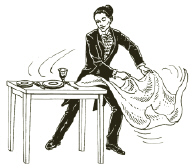Which of the following best explains the performer’s success at leaving all the dinnerware on the table?
 a. the inertia of the dinnerware c. the placement of the dinnerware b. the large mass of the tablecloth d. the rough material of the tablecloth

5.

A toy cart that has a weight of 10 N moves with a constant velocity of 2 m/s to the right on a horizontal table. According to Newton’s laws of motion, which of the following statements is correct? (MCAS 2009)
 a. The table exerts a force of 10 N upward on the toy cart. b. The toy cart exerts a force of 10 N upward on the table. c. The toy cart exerts a force of 2 N downward on the table. d. The table exerts a force of 2 N to the right on the toy cart.

6.

Which of the following will always change when an object accelerates? (MCAS 2010)
 a. mass c. velocity b. potential energy d. weight

7.

A car has an oil drip. As the car moves, it drips oil at a regular rate, leaving a trail of spots on the road. Which of the following diagrams of the car’s trail of spots shows the car continuously slowing down? (MCAS 2009)
 a.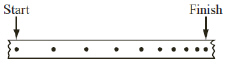c.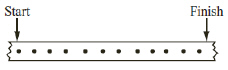b.d.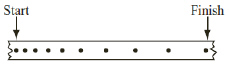8.

The table below shows the time it takes four cars to go from 0 to 60 km/h.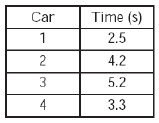Based on the information given, which of the following quantities can be compared for the four cars? (MCAS 2010)
 a. average acceleration c. stopping distance b. instantaneous speed d. stopping time

9.

Two students are in-line skating. The diagram below shows student S pushing student T. Student S has a mass of 60 kg,and student T has a mass of 40 kg.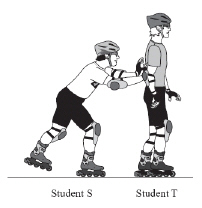Student S is pushing student T in a straight line with a force equal to the friction on student T’s skates. Which of the following must happen if student S continues to push student T with the same force? (MCAS 2011)
 a. Student T will lose his balance. b. Student T will travel in a circle. c. Student T will move at a constant velocity. d. Student T will move at a constant acceleration.

10.

A satellite in a circular orbit around Earth has a constant speed but not a constant velocity. Which of the following statements best explains why the satellite’s velocity is not constant? (MCAS 2013)
 a. The radius of the satellite’s orbit is too large. b. The force on the satellite’s mass is constantly decreasing. c. The magnitude of the satellite’s momentum is too large. d. The direction of the satellite’s motion is constantly changing.

11.

The diagram below shows a ball tied to a string. A student is swinging the ball in a horizontal circle.If the student releases the string, in which direction will the ball travel? (MCAS 2013)
 a. direction W c. direction Y b. direction X d. direction Z

Problems

12.

The illustration below shows a car slowing down.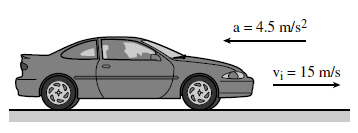The car was initially traveling at 15 m/s. The car slows with a negative acceleration of 4.5 m/s2. How long does it take the car to slow to a final velocity of 4.0 m/s? (Modified MCAS 2004)

13.

A 1500 kg car increases its speed by 2 m/s for each second of travel. What is the net force acting on the car? (Modified MCAS 2005)

14.

What is the mass of an object weighing 63 N on Earth? (Modified MCAS 2007)

15.

How long will it take a car to accelerate from 20 m/s to 26 m/s at a rate of 1.4 m/s2? (Modified MCAS 2007)

16.

A 1500 kg car has an applied forward force of 5000 N and experiences an air resistance of 1250 N.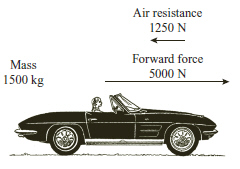What is the car’s acceleration? (Modified MCAS 2008)

17.

A bicycle rider is traveling at 7 m/s. During an 8 s period, the bicycle rider then slows down with a constant acceleration to a speed of 3 m/s. How far does the bicycle rider travel during the 8 s? (Modified MCAS 2008)

18.

A ball has a mass of 0.1 kg and an initial velocity of 20 m/s. The ball is given an acceleration of 30 m/s2 for 5 s. What is the net force on the ball during acceleration? (Modified MCAS 2009)

19.

A rock is dropped from a window 5 m above the ground. The rock hits the ground 1.0 s later with a speed of 10 m/s. What is the average speed of the rock during this time? (Modified MCAS 2012)

Essay

20.

The figure below is a graph of net force vs. the acceleration of an object. (MCAS 2006)a.       Use the graph to determine the mass of the object. Show your calculations and include units in your answer.
b.       What acceleration will the object have if the net force is 50 N and the trend shown by the graph continues? Show your calculations and include units in your answer.
c.       On the grid in your Student Answer Booklet, draw a graph of force vs. acceleration if the mass of the object is halved and the object is subjected to the same net forces. Label the axes on your graph and be sure to include units. Label this graph “c.”
d.       On the same axes that you used in part (c), draw a graph of force vs. acceleration if the mass of the object is doubled and the object is subjected to the same net forces. Label this graph “d.”

21.

A book is on a table. A student pushes it for a short time. Initially the book moves, but then it comes to a complete stop. (MCAS 2007)

a.       Identify the forces acting on the book before it is pushed. You may include a labeled diagram in your answer.

b.       Explain why the book moves and then comes to a complete stop. Use the laws of physics in your answer.

c.       The student wants the book to move at a constant speed in one direction. Describe the physical conditions needed for this to occur.

22.

An elastic cord made for bungee jumping is being tested. A weight of 800 N is attached to one end of the bungee cord. Then the weight is released from a tall tower and it moves downward. When the elastic cord is fully extended, it exerts an opposing force of 900 N on the weight. (MCAS 2008)

a.       Draw and label a force diagram for this situation.

b.       Calculate the net force on the weight. Show your calculations and include units in your answer.

c.       Explain what would happen if the elastic cord exerted a maximum force of only 700 N on the weight.